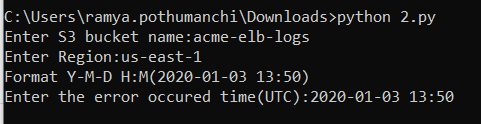python boto3 s3 aws 1 year, 11 months ago
import boto3 import datetime from datetime import datetime,timedelta,date import os client = boto3.client('s3') s3 = boto3.resource('s3') iam = boto3.resource('iam') account_id = iam.CurrentUser().arn.split(':') buckername=input("Enter S3 bucket name:") region=input("Enter Region:") now=input("Format Y-M-D H:M(2020-01-03 13:50)\nEnter the error occured time(UTC):") s=datetime.strptime(now,'%Y-%m-%d %H:%M') sdate_time= s - timedelta(minutes = 10) edate_time= s + timedelta(minutes = 10) s_time=sdate_time.strftime('%Y-%m-%d %H:%M') e_time=edate_time.strftime('%Y-%m-%d %H:%M') y= str(s_time[:4]) m= str(s_time[5:7]) d=str(s_time[8:10]) prefix= "AWSLogs/"+account_id+"/elasticloadbalancing/"+region+"/"+y+"/"+m+"/"+d objects = client.list_objects(Bucket=buckername,Prefix=prefix+'/', Delimiter='/') i=1 for o in objects["Contents"]: key=(o["Key"]) time=str(o["LastModified"]) cd=time[:16] tt = datetime.strptime(str(time[:10]),'%Y-%m-%d') d1 = datetime.strptime(s_time, '%Y-%m-%d %H:%M') d2 = datetime.strptime(e_time, '%Y-%m-%d %H:%M') c1 = datetime.strptime(str(cd), '%Y-%m-%d %H:%M') if(d1<=c1<=d2): s3.meta.client.download_file(Bucket=buckername,Key=key,Filename='file_'+str(i)+'.gz') i=i+1
1090Instructions

Before using this script, you need to set up AWS CLI first as below:

ubuntu@ip-172-31-46-2:~\$ aws configure
AWS Access Key ID [None]: <access_key>
AWS Secret Access Key [None]: <secret_key>
Default region name [None]: <region>
Default output format [None]: <eg:JSON>